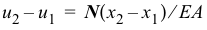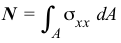# 3.1 – THEORY OF AXIAL MEMBERS

 In the questions below, select the correct answer. NameEmail1) Axial strain is uniform across a nonhomogeneous cross section. True False2) Axial stress is uniform across a nonhomogeneous cross section. True False3) The formulacan be used for finding the stress on a cross section of a tapered axial member. True False4) The formulacan be used for finding the deformation of a segment of a tapered axial member. True False5) The formulacan be used for finding the stress on a cross section of an axial member subjected to distributed forces. True False6) The formulacan be used for finding the deformation of a segment of an axial member subjected to distributed forces. True False7) The equationcannot be used for nonlinear materials. True False8) The equationcan be used for a nonhomogeneous cross section. True False9) External axial forces must be colinear and pass through the centroid of a homogeneous cross section for no bending to occur. True False10) Internal axial forces jump by the value of the concentrated external axial force at a section. True FalseDo you have any comments or suggestions? Please send them to author@madhuvable.org